Draw curved geometry
This section describes how to add circular geometry to your drawing. There are a number of different ways to draw circles and arcs.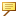Creo Elements/Direct Annotation creates arcs in the counterclockwise direction.
1. Click Geometry and then, in the Draw group, click the arrow next to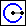Circle.
2. Choose one of the following:
To create a circle with center and radius,
1. ClickCenter & Radius in the Circle section. The Circle Center Radius dialog box opens.
2. Specify the center point of the circle in one of the following ways:
Click a point.
Enter coordinates in the user input line.
3. Specify the radius of the circle in one of the following ways:
Specify a point on the circumference:
Click a point on the circumference.
Enter the coordinates of a point on the circumference in the user input line.
Enter the radius in the user input line.
To create a circle concentric to an existing circle or arc,
1. Click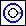Concentric in the Circle section. The Circle Concentric dialog box opens.
2. Click the existing circle or arc.
3. Specify the offset of the new circle in one of the following ways:
Specify a point on the circumference:
Click a point on the circumference.
Enter the coordinates of a point on the circumference in the user input line.
Enter the offset of the new circle in the user input line. (A positive value increases the radius, a negative value decreases it.)
To create a circle with three points,
1. Click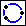3 Points in the Circles section. The Circle with 3 Pos dialog box opens.
2. Specify three circumference points in one of the following ways:
Click the points on the screen.
Enter the coordinates of the points in the user input line.
To create a circle with a given diameter,
1. Click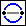Diameter in the Circles section. The Circle by Diameter dialog box opens.
2. Specify the two diameter end points for the circle in one of the following ways:
Click the points on the screen.
Enter the coordinates of the points in the user input line.
To create a circle by radius and two tangent elements,
You can draw a circle of given radius tangential to two existing elements. The elements of tangency can be lines, arcs, or circles.
1. Click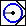Tangent by 2 in the Circles section. The Circle Tan by 2 dialog box opens.
2. Click approximate tangent points on two elements.
3. Specify the radius for the circle in one of the following ways:
Click a point on the circumference.
Enter the radius in the user input line.
Creo Elements/Direct Annotation calculates the best possible solution for the circle.
3. To create arcs,
a. Click Geometry and then, in the Draw group, click the arrow next to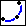Arc.
b. Choose one of the following:
To create an arc by the center,
1. Click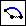Center & 2 Points in the Arc section. The Arc Center dialog box opens.
2. Specify the center point for the arc in one of the following ways:
Click the point on the screen.
Enter the coordinates in the user input line.
3. Specify the position to begin the arc in one of the following ways:
Click the point on the screen.
Enter the coordinates in the user input line.
4. Specify the end point of the arc as above.
To create an arc concentric to an existing arc,
1. Click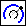Concentric in the Arc section. The Arc Concentric dialog box opens.
2. Click the existing arc.
3. Specify the offset of the new arc in one of the following ways:
Specify a point on the circumference:
Click a point on the circumference.
Enter the coordinates of a point on the circumference in the user input line.
Enter the offset of the new arc in the user input line. (A positive value increases the radius, a negative value decreases it.)
To create an arc by specifying three points,
1. Click3 Points in the Arc section. The Arc 3 Pos dialog box opens.
2. Specify the begin point for the arc in one of the following ways:
Click the point.
Enter the coordinates in the user input line.
3. Specify the end point of the arc as above.
4. Specify the third point, as above, that defines the circumference of the arc.
To create an arc by specifying its diameter,
1. Click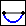Diameter in the Arc section. The Arc Diameter dialog box opens.
2. Specify the two diameter end points for the arc in one of the following ways:
Click the points on the screen.
Enter the coordinates of the points in the user input line.
To create a generic arc,
1. Click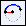Center, Radius & 2 Points in the Arc section. The Arc Generic dialog box opens.
2. Specify the center point for the arc in one of the following ways:
Click the point on the screen.
Enter the coordinates in the user input line.
3. Specify the radius for the arc in one of the following ways:
Enter the radius in the user input line.
Specify a point on the arc:
Click a point.
Enter the coordinates in the user input line.
4. Specify the position to begin the arc in one of the following ways:
Enter an angle (relative to the positive x-axis).
Click a point.
Enter the coordinates in the user input line.
5. Specify the end point of the arc as above.
To create a smooth arc,
You can draw an arc with a smooth connection to an existing open element.
1. Click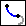Smooth in the Arc section. The Arc Smooth dialog box opens.
2. Click an end point of the open element for the arc to be smooth to.
3. Specify the end point of the arc in one of the following ways:
Click the point.
Enter the coordinates in the user input line.
4. Continue creating circles or arcs or click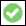to complete the operation.
The circles or arcs drawn follow the movement of the cursor until their end points are defined.